### Bunny Tunes in Hi-Def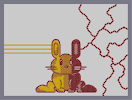Hover over the thumbnail for a full-size version.

Author darkcrusader author:darkcrusader cheezburger darkcrusader featured gold mines n-art rated 2011-09-23 2011-10-30 2 by 28 people. \$Bunny Tunes in Hi-Def#darkcrusader#n-art#00000000000000000000000000000000000000000000000000000000000000000000000000000000000000000000000000000000000000000000000000000000000000000000000000000000000000000000000000000000000000000000000000000000000000000000000000000000000000000000000000000000000000000000000000000000000000000000000000000000000000000000000000000000000000000000000000000000000000000000000000000000000000000000000000000000000000000000000000000000000000000000000000000000000000000000000000000000000000000000000000000000000000000000000000000000000000000000000000000000000000000000000000000000000000000000000000000000000000000000000000000000000000000000000000000000000000000000000000000000000000000000000000000000000000000000000000000000000000000|0^24,270!0^30,270!0^36,270!0^42,270!0^48,270!0^60,270!0^54,270!0^66,270!0^72,270!0^78,270!0^84,270!0^90,270!0^96,270!0^102,270!0^108,270!0^114,270!0^120,270!0^126,270!0^132,270!0^138,270!0^144,270!0^150,270!0^156,270!0^162,270!0^168,270!0^174,270!0^180,270!0^186,270!0^192,270!0^198,270!0^204,270!0^210,270!0^216,270!0^222,270!0^228,270!0^234,270!0^240,270!0^246,270!0^252,270!0^258,270!0^264,270!0^270,270!0^276,270!0^282,270!0^288,270!0^294,270!0^300,270!0^306,270!0^24,294!0^30,294!0^36,294!0^42,294!0^48,294!0^54,294!0^60,294!0^66,294!0^72,294!0^84,294!0^78,294!0^90,294!0^96,294!0^102,294!0^108,294!0^114,294!0^120,294!0^126,294!0^132,294!0^138,294!0^144,294!0^150,294!0^156,294!0^162,294!0^168,294!0^180,294!0^174,294!0^186,294!0^192,294!0^198,294!0^204,294!0^210,294!0^216,294!0^222,294!0^228,294!0^234,294!0^240,294!0^246,294!0^252,294!0^258,294!0^264,294!0^270,294!0^276,294!0^282,294!0^288,294!0^294,294!0^300,294!0^306,294!0^312,294!0^312,270!0^312,318!0^306,318!0^300,318!0^294,318!0^288,318!0^282,318!0^276,318!0^270,318!0^264,318!0^258,318!0^252,318!0^246,318!0^240,318!0^234,318!0^228,318!0^222,318!0^216,318!0^210,318!0^204,318!0^198,318!0^192,318!0^186,318!0^180,318!0^174,318!0^168,318!0^162,318!0^156,318!0^150,318!0^144,318!0^138,318!0^132,318!0^126,318!0^120,318!0^114,318!0^108,318!0^102,318!0^96,318!0^90,318!0^84,318!0^78,318!0^72,318!0^66,318!0^60,318!0^54,318!0^48,318!0^42,318!0^36,318!0^30,318!0^24,318!12^312,318!12^312,312!12^312,306!12^312,300!12^312,294!12^312,288!12^312,282!12^312,276!12^324,240!12^315,251!12^312,265!12^312,270!12^313,260!12^314,255!12^321,242!12^319,246!12^317,249!12^337,349!12^325,343!12^314,325!12^318,334!12^332,347!12^330,346!12^323,341!12^320,338!12^316,330!12^331,237!12^329,238!12^349,241!12^338,237!12^344,238!12^348,240!12^336,238!12^352,244!12^356,251!12^358,260!12^360,282!12^360,276!12^360,267!12^360,272!12^357,256!12^354,247!12^359,267!12^360,288!12^360,300!12^360,294!12^360,306!12^360,312!12^360,318!12^360,324!12^356,337!12^359,332!12^396,324!12^375,328!12^418,330!12^408,325!12^386,325!12^365,336!12^360,341!12^431,341!12^369,332!12^381,327!12^390,324!12^402,325!12^425,335!12^429,340!12^425,333!12^413,327!12^436,337!12^434,333!12^432,324!12^432,318!12^432,312!12^432,306!12^432,294!12^432,300!12^432,288!12^432,288!12^432,276!12^432,282!12^432,270!12^432,264!12^432,330!12^480,264!12^480,270!12^480,276!12^480,282!12^480,288!12^480,294!12^480,300!12^480,306!12^480,312!12^456,348!12^474,335!12^466,343!12^480,323!12^468,241!12^436,249!12^476,249!12^446,241!12^457,238!12^440,244!12^453,239!12^473,245!12^462,238!12^479,261!12^479,258!12^478,253!12^432,260!12^434,255!12^480,318!12^478,331!12^479,328!12^470,341!12^460,346!12^464,345!12^475,334!12^472,340!12^312,372!12^312,420!12^396,444!12^480,420!12^480,372!12^486,396!12^306,396!12^360,384!12^366,390!12^372,384!12^372,360!12^360,360!12^366,354!12^362,356!12^370,356!12^369,388!12^372,384!12^372,378!12^372,372!12^372,366!12^360,378!12^360,372!12^360,366!12^362,388!12^366,384!12^366,378!12^366,372!12^368,369!12^420,360!12^432,360!12^432,378!12^432,384!12^420,384!12^426,390!12^426,354!12^423,356!12^430,356!12^430,388!12^423,388!12^420,378!12^420,372!12^420,366!12^432,366!12^432,372!12^432,378!12^444,438!12^348,438!12^325,358!12^308,383!12^307,409!12^329,433!12^372,443!12^422,443!12^465,431!12^467,359!12^455,353!12^337,354!12^332,355!12^318,364!12^475,365!12^463,356!12^472,362!12^485,383!12^473,427!12^408,402!12^384,402!12^396,396!12^388,398!12^390,397!12^408,399!12^405,397!12^402,396!12^396,408!12^401,407!12^406,405!12^385,404!12^391,406!12^404,403!12^398,406!12^456,435!12^432,441!12^414,444!12^408,444!12^402,444!12^390,444!12^384,444!12^378,444!12^417,444!12^428,443!12^436,441!12^441,440!12^449,437!12^461,433!12^471,428!12^477,423!12^484,377!12^479,368!12^369,442!12^363,442!12^355,440!12^343,437!12^335,434!12^324,431!12^320,427!12^316,424!12^311,418!12^308,415!12^307,408!12^306,402!12^306,393!12^307,387!12^309,380!12^310,375!12^316,368!12^321,361!12^486,390!12^486,403!12^482,415!12^484,411!12^485,408!12^312,468!12^318,444!12^313,479!12^312,474!12^312,465!12^312,460!12^313,456!12^316,449!12^315,453!12^322,439!12^324,435!12^467,433!12^470,438!12^481,479!12^482,478!12^482,473!12^482,468!12^482,464!12^482,460!12^480,451!12^481,455!12^478,447!12^476,444!12^473,440!12^469,438!12^312,492!12^480,492!12^504,492!12^288,492!12^300,486!12^492,486!12^504,522!12^510,510!12^534,510!12^576,534!12^570,552!12^468,552!12^462,546!12^468,528!12^282,510!12^288,522!12^258,510!12^216,534!12^228,552!12^324,552!12^330,546!12^324,528!12^234,552!12^240,552!12^246,552!12^252,552!12^258,552!12^264,552!12^270,552!12^282,552!12^276,552!12^288,552!12^300,552!12^294,552!12^306,552!12^312,552!12^318,552!12^474,552!12^480,552!12^486,552!12^492,552!12^504,552!12^498,552!12^510,552!12^516,552!12^522,552!12^534,552!12^528,552!12^540,552!12^546,552!12^558,552!12^552,552!12^564,552!12^329,550!12^463,550!12^463,537!12^462,542!12^466,533!12^328,535!12^329,540!12^326,533!12^485,488!12^499,488!12^509,499!12^510,507!12^510,504!12^520,511!12^516,514!12^525,511!12^529,510!12^539,511!12^545,513!12^551,515!12^556,517!12^560,520!12^565,524!12^570,528!12^574,532!12^575,549!12^578,544!12^578,539!12^222,550!12^220,547!12^218,542!12^217,540!12^232,518!12^243,513!12^222,525!12^273,514!12^283,500!12^294,487!12^308,489!12^305,488!12^218,530!12^227,521!12^238,515!12^249,511!12^251,511!12^267,513!12^263,511!12^282,506!12^285,497!12^291,490!12^276,516!12^282,519!12^283,517!12^283,515!12^324,504!12^336,534!12^354,552!12^384,552!12^366,528!12^384,534!12^408,534!12^426,528!12^438,552!12^456,534!12^468,504!12^315,486!12^322,500!12^319,495!12^317,491!12^326,508!12^329,514!12^333,519!12^335,526!12^343,548!12^338,541!12^388,543!12^387,548!12^387,538!12^380,531!12^372,527!12^376,528!12^407,538!12^340,545!12^337,540!12^326,525!12^329,521!12^348,551!12^360,552!12^366,552!12^372,552!12^378,552!12^362,529!12^364,525!12^365,520!12^362,481!12^367,496!12^367,510!12^365,517!12^367,505!12^367,500!12^367,491!12^366,488!12^364,485!12^360,480!12^432,480!12^425,497!12^427,487!12^428,484!12^425,492!12^424,502!12^424,507!12^425,512!12^428,521!12^428,517!12^431,528!12^430,524!12^427,516!12^415,528!12^406,544!12^411,530!12^419,528!12^409,550!12^414,552!12^420,552!12^426,552!12^432,552!12^450,548!12^445,550!12^452,543!12^454,537!12^474,493!12^479,484!12^476,490!12^471,500!12^473,496!12^466,508!12^463,515!12^457,528!12^459,523!12^461,520!12^464,513!12^468,528!12^463,524!12^486,294!12^492,294!12^498,294!12^504,288!12^510,282!12^516,282!12^522,276!12^528,264!12^528,270!12^552,264!12^546,258!12^534,258!12^540,258!12^552,246!12^552,252!12^558,240!12^564,234!12^570,228!12^570,222!12^576,216!12^564,216!12^558,216!12^552,210!12^546,204!12^582,210!12^582,204!12^588,198!12^612,174!12^618,168!12^624,162!12^624,174!12^630,174!12^636,180!12^642,180!12^648,186!12^654,192!12^660,198!12^666,198!12^678,192!12^672,192!12^684,186!12^690,186!12^696,192!12^702,192!12^708,186!12^714,180!12^720,174!12^726,174!12^732,168!12^738,168!12^744,162!12^750,168!12^756,168!12^762,174!12^768,174!12^558,270!12^564,270!12^570,276!12^576,282!12^582,288!12^588,288!12^594,294!12^600,300!12^600,306!12^606,312!12^600,318!12^600,324!12^606,330!12^612,336!12^618,336!12^624,342!12^630,336!12^636,336!12^642,330!12^648,336!12^654,336!12^660,342!12^666,348!12^672,348!12^678,354!12^672,360!12^672,366!12^678,372!12^678,378!12^672,384!12^648,324!12^648,318!12^654,312!12^654,306!12^648,300!12^642,294!12^642,288!12^648,282!12^648,276!12^654,270!12^654,264!12^660,258!12^666,258!12^672,264!12^678,264!12^684,258!12^690,258!12^696,264!12^708,270!12^702,270!12^714,276!12^720,270!12^726,270!12^732,264!12^738,258!12^744,252!12^744,246!12^750,240!12^762,234!12^756,234!12^768,228!12^630,156!12^630,150!12^636,144!12^642,144!12^648,138!12^654,138!12^660,144!12^666,138!12^672,132!12^678,126!12^678,120!12^672,114!12^672,108!12^666,102!12^666,96!12^660,90!12^654,90!12^648,84!12^642,78!12^642,72!12^648,66!12^654,60!12^654,54!12^660,48!12^666,48!12^672,42!12^678,42!12^684,36!12^690,36!12^696,30!12^696,24!12^546,198!12^540,192!12^534,186!12^528,180!12^528,174!12^522,168!12^522,162!12^528,156!12^528,150!12^534,144!12^534,138!12^540,132!12^540,126!12^534,120!12^528,114!12^522,108!12^522,102!12^516,96!12^510,90!12^504,84!12^498,84!12^492,78!12^486,72!12^480,66!12^480,60!12^474,54!12^474,48!12^480,42!12^480,36!12^684,384!12^690,390!12^696,384!12^702,384!12^708,378!12^714,378!12^720,372!12^726,366!12^720,384!12^726,390!12^732,390!12^738,396!12^744,402!12^744,408!12^750,414!12^744,420!12^744,426!12^738,432!12^744,438!12^744,444!12^738,450!12^732,456!12^726,462!12^720,462!12^714,468!12^714,474!12^708,480!12^714,486!12^714,492!12^720,498!12^720,504!12^726,510!12^726,516!12^732,522!12^738,528!12^744,528!12^750,534!12^756,534!12^768,528!12^762,528!12^594,186!12^588,192!12^600,180!12^606,180!12^732,360!12^738,360!12^744,354!12^750,348!12^750,342!12^756,336!12^762,330!12^768,330!12^666,390!12^666,396!12^672,402!12^672,408!12^678,414!12^678,420!12^684,426!12^678,432!12^678,438!12^672,444!12^666,450!12^666,456!12^660,462!12^654,468!12^648,468!12^642,474!12^636,486!12^636,480!12^630,492!12^624,498!12^624,504!12^618,510!12^618,516!12^624,522!12^630,528!12^636,534!12^636,540!12^630,546!12^630,552!12^624,558!12^618,564!12^618,570!12^624,576!12^500,292!12^507,284!12^518,280!12^525,273!12^530,260!12^548,254!12^550,262!12^554,267!12^567,272!12^573,278!12^577,283!12^580,286!12^592,291!12^597,298!12^603,309!12^604,315!12^602,327!12^610,334!12^621,339!12^627,339!12^639,331!12^645,332!12^656,337!12^662,344!12^658,339!12^638,334!12^645,329!12^652,316!12^652,304!12^645,298!12^645,285!12^651,274!12^658,260!12^669,260!12^681,261!12^695,260!12^699,268!12^712,273!12^718,273!12^728,268!12^736,260!12^740,256!12^747,242!12^753,237!12^763,232!12^759,171!12^747,166!12^741,165!12^729,171!12^716,178!12^710,184!12^705,190!12^693,188!12^682,188!12^669,195!12^657,195!12^650,189!12^645,184!12^634,178!12^622,172!12^620,166!12^626,160!12^615,172!12^609,177!12^597,183!12^589,191!12^590,191!12^585,202!12^579,214!12^572,220!12^567,232!12^561,237!12^554,244!12^676,351!12^675,357!12^675,369!12^669,388!12^681,381!12^676,380!12^688,388!12^694,387!12^705,381!12^716,380!12^716,376!12^724,368!12^729,363!12^742,357!12^747,352!12^753,339!12^759,333!12^718,384!12^723,388!12^734,393!12^741,398!12^748,411!12^747,419!12^741,429!12^741,435!12^742,447!12^735,453!12^730,460!12^717,466!12^711,476!12^711,484!12^717,496!12^722,506!12^730,520!12^735,524!12^746,531!12^760,531!12^669,400!12^675,411!12^681,422!12^682,429!12^674,442!12^668,449!12^662,460!12^657,465!12^645,472!12^638,478!12^633,489!12^627,495!12^621,508!12^621,520!12^627,525!12^634,532!12^633,544!12^626,555!12^621,562!12^621,573!12^632,147!12^645,141!12^657,141!12^663,141!12^669,134!12^675,129!12^675,118!12^668,105!12^664,93!12^651,88!12^644,82!12^644,69!12^651,62!12^657,50!12^669,45!12^682,39!12^694,34!12^486,30!12^486,24!12^483,34!12^477,46!12^478,58!12^484,70!12^489,74!12^495,82!12^507,87!12^513,93!12^519,99!12^525,112!12^531,117!12^538,124!12^537,136!12^530,147!12^524,160!12^525,172!12^531,184!12^537,189!12^545,196!12^549,209!12^556,214!12^567,219!12^324,276!12^348,276!12^324,318!12^348,318!12^336,258!12^336,330!12^456,330!12^468,318!12^444,318!12^444,276!12^468,276!12^456,258!12^444,312!12^444,300!12^444,294!12^444,282!12^468,282!12^468,288!12^468,300!12^468,294!12^468,306!12^468,312!12^348,312!12^348,306!12^348,300!12^348,294!12^348,294!12^348,288!12^348,282!12^324,282!12^324,294!12^324,288!12^324,300!12^324,306!12^324,312!12^328,325!12^331,328!12^326,322!12^341,329!12^345,325!12^346,322!12^328,263!12^344,263!12^341,261!12^331,260!12^326,267!12^325,273!12^347,273!12^346,266!12^445,270!12^453,259!12^449,262!12^446,266!12^468,271!12^468,267!12^391,542!12^397,542!12^402,542!12^446,322!12^448,325!12^451,328!12^467,322!12^464,326!12^460,328!12^463,260!12^467,264!12^336,402!12^330,402!12^318,402!12^324,402!12^312,402!12^300,402!12^288,402!12^294,402!12^276,402!12^282,402!12^336,414!12^342,414!12^330,414!12^324,414!12^318,414!12^312,414!12^306,414!12^300,414!12^294,414!12^288,414!12^486,402!12^492,402!12^498,402!12^504,402!12^516,402!12^510,402!12^486,414!12^492,414!12^498,414!12^504,414!0^318,264!0^318,270!0^318,276!0^318,282!0^318,288!0^318,294!0^318,300!0^318,306!0^318,312!0^318,324!0^318,318!0^324,330!0^330,336!0^336,342!0^342,342!0^336,336!0^342,336!0^348,330!0^354,324!0^354,312!0^354,318!0^354,306!0^354,294!0^354,300!0^354,288!0^354,282!0^354,276!0^354,264!0^354,270!0^348,258!0^324,258!0^324,252!0^330,252!0^336,252!0^342,252!0^348,252!0^330,246!0^342,246!0^342,246!0^336,246!0^348,336!0^348,348!0^348,342!0^342,354!0^342,348!0^348,354!0^348,360!0^342,360!0^336,360!0^330,360!0^330,366!0^324,372!0^318,378!0^318,384!0^318,396!0^312,390!0^318,390!0^324,384!0^324,378!0^324,366!0^318,372!0^312,396!0^342,408!0^336,408!0^330,408!0^324,408!0^318,408!0^312,408!0^342,402!0^342,396!0^336,396!0^330,396!0^324,396!0^324,390!0^330,390!0^330,384!0^330,378!0^330,372!0^336,366!0^336,372!0^336,378!0^336,384!0^336,390!0^342,390!0^342,384!0^342,378!0^342,366!0^342,372!0^354,330!0^324,336!0^318,258!0^324,246!0^354,342!0^354,348!0^354,348!0^354,354!0^354,360!0^354,366!0^354,372!0^354,378!0^354,384!0^354,390!0^354,408!0^354,402!0^354,396!0^354,414!0^348,414!0^348,402!0^348,408!0^348,396!0^348,390!0^348,384!0^348,378!0^348,372!0^348,372!0^348,366!0^324,420!0^330,420!0^336,420!0^342,420!0^348,420!0^354,420!0^330,426!0^342,426!0^348,426!0^354,426!0^318,420!0^336,426!0^342,432!0^354,432!0^354,432!0^348,432!0^366,432!0^360,432!0^360,426!0^360,414!0^360,414!0^360,420!0^360,408!0^360,402!0^360,396!0^366,396!0^366,402!0^366,408!0^366,414!0^366,420!0^366,426!0^372,438!0^372,432!0^372,426!0^372,420!0^372,414!0^372,402!0^372,408!0^372,396!0^378,390!0^378,384!0^378,378!0^378,366!0^378,372!0^378,360!0^378,402!0^378,396!0^378,408!0^378,414!0^378,420!0^378,432!0^378,426!0^378,438!0^378,354!0^378,348!0^372,348!0^366,348!0^360,348!0^372,342!0^366,342!0^372,336!0^378,336!0^384,330!0^390,330!0^402,330!0^396,336!0^396,330!0^390,336!0^384,336!0^384,342!0^378,342!0^408,390!0^408,378!0^408,384!0^408,372!0^408,366!0^408,360!0^408,354!0^408,348!0^408,342!0^408,330!0^408,336!0^402,342!0^402,342!0^402,336!0^396,342!0^390,342!0^384,348!0^384,354!0^384,366!0^384,360!0^384,378!0^384,372!0^384,384!0^384,390!0^390,390!0^402,390!0^396,390!0^396,384!0^390,384!0^390,378!0^402,378!0^396,384!0^396,378!0^390,372!0^390,366!0^396,366!0^396,372!0^402,372!0^402,366!0^402,384!0^402,360!0^402,354!0^402,348!0^396,354!0^396,360!0^390,360!0^390,354!0^390,348!0^396,348!0^384,414!0^390,414!0^396,414!0^402,414!0^408,414!0^408,420!0^408,420!0^408,426!0^408,432!0^408,438!0^396,432!0^402,438!0^396,438!0^390,438!0^384,438!0^384,432!0^396,432!0^384,426!0^384,420!0^396,420!0^390,426!0^390,420!0^390,432!0^396,426!0^402,426!0^402,420!0^402,438!0^402,432!0^408,450!0^408,450!0^408,456!0^408,462!0^408,474!0^408,468!0^408,480!0^408,486!0^408,492!0^408,498!0^408,504!0^408,510!0^408,516!0^408,522!0^408,528!0^402,534!0^396,534!0^390,528!0^384,522!0^372,522!0^378,522!0^372,516!0^378,510!0^372,510!0^372,498!0^372,504!0^372,492!0^372,486!0^372,480!0^366,474!0^378,516!0^384,516!0^390,516!0^390,522!0^402,522!0^402,528!0^396,528!0^396,522!0^396,516!0^402,516!0^402,510!0^390,510!0^384,510!0^396,510!0^378,480!0^390,480!0^396,480!0^402,480!0^378,492!0^378,498!0^378,504!0^384,504!0^390,498!0^396,492!0^402,486!0^396,498!0^402,504!0^390,492!0^390,486!0^330,450!0^330,456!0^330,462!0^330,468!0^330,474!0^342,474!0^342,468!0^342,462!0^342,456!0^342,450!0^336,462!0^354,450!0^360,450!0^366,450!0^360,456!0^360,462!0^360,468!0^360,474!0^354,474!0^384,450!0^384,456!0^384,462!0^378,486!0^384,474!0^384,486!0^384,480!0^384,492!0^384,498!0^396,504!0^390,504!0^402,498!0^402,492!0^396,486!0^402,474!0^396,474!0^390,474!0^390,468!0^390,462!0^390,456!0^390,450!0^396,450!0^402,450!0^402,468!0^390,468!0^402,468!0^396,462!0^396,468!0^402,462!0^402,456!0^396,462!0^396,456!0^384,474!0^384,468!0^378,474!0^372,474!0^372,468!0^384,468!0^378,468!0^378,462!0^378,462!0^372,462!0^378,456!0^378,450!0^372,450!0^372,456!0^366,456!0^366,468!0^366,462!0^354,468!0^360,462!0^354,456!0^354,462!0^348,444!0^342,444!0^336,444!0^330,444!0^330,438!0^324,450!0^324,444!0^324,456!0^324,462!0^324,468!0^318,468!0^318,462!0^318,456!0^336,450!0^336,456!0^336,468!0^336,474!0^348,474!0^348,468!0^348,462!0^348,456!0^348,450!0^366,480!0^384,528!0^396,540!0^390,534!0^384,414!0^384,408!0^354,444!0^324,474!0^318,474!0^324,480!0^324,486!0^318,480!0^330,480!0^330,486!0^330,492!0^330,498!0^324,492!0^336,480!0^336,486!0^342,486!0^342,480!0^348,486!0^348,480!0^354,480!0^354,492!0^354,486!0^336,498!0^336,498!0^336,492!0^342,492!0^342,498!0^348,498!0^348,492!0^354,498!0^360,498!0^360,492!0^360,504!0^360,510!0^354,510!0^354,504!0^348,510!0^348,504!0^342,504!0^342,510!0^336,510!0^336,504!0^330,504!0^342,516!0^348,516!0^354,516!0^360,516!0^336,516!0^342,522!0^342,528!0^348,522!0^360,522!0^354,522!0^354,528!0^348,528!0^342,534!0^348,534!0^354,534!0^360,534!0^372,534!0^366,534!0^378,540!0^378,546!0^372,546!0^366,546!0^360,546!0^354,546!0^348,540!0^360,540!0^354,540!0^366,540!0^372,540!0^318,516!0^318,522!0^324,516!0^318,510!0^318,504!0^312,498!0^306,498!0^300,498!0^300,492!0^294,498!0^294,504!0^288,510!0^294,516!0^294,510!0^288,504!0^300,504!0^306,504!0^312,504!0^312,510!0^306,510!0^300,510!0^300,516!0^306,516!0^312,516!0^312,522!0^306,522!0^300,522!0^294,522!0^318,528!0^318,534!0^324,540!0^324,546!0^318,546!0^318,540!0^312,546!0^312,540!0^312,534!0^312,528!0^306,528!0^306,540!0^306,534!0^300,534!0^300,534!0^300,528!0^294,528!0^306,546!0^300,546!0^300,540!0^294,540!0^294,534!0^288,534!0^288,528!0^288,540!0^294,546!0^288,546!0^282,546!0^282,540!0^282,534!0^282,528!0^282,522!0^276,522!0^270,522!0^270,522!0^258,522!0^264,522!0^258,516!0^276,528!0^270,540!0^276,540!0^276,534!0^276,546!0^270,546!0^270,534!0^270,528!0^264,534!0^264,528!0^258,528!0^258,534!0^258,540!0^258,546!0^264,546!0^264,540!0^252,546!0^252,540!0^252,534!0^252,528!0^252,522!0^252,516!0^246,522!0^240,522!0^234,528!0^228,528!0^222,534!0^222,540!0^228,540!0^228,546!0^240,546!0^246,546!0^234,546!0^234,534!0^228,534!0^228,540!0^234,540!0^240,540!0^246,540!0^246,534!0^246,528!0^240,528!0^240,534!12^336,318!12^342,312!12^342,324!12^330,324!12^336,306!12^342,300!12^342,288!12^330,312!12^462,312!12^450,324!12^462,324!12^456,306!12^462,300!12^462,288!12^222,534!12^228,540!12^234,546!12^222,546!12^234,534!12^228,528!12^240,540!12^246,546!12^252,540!12^258,546!12^270,546!12^342,540!12^348,546!12^354,540!12^360,546!12^348,534!12^372,546!12^408,540!0^336,324!0^342,318!0^330,318!0^336,312!0^342,306!0^330,306!0^330,300!0^336,300!0^336,294!0^342,294!0^330,294!0^336,288!0^330,288!0^330,282!0^336,282!0^342,282!0^330,276!0^336,276!0^342,276!0^336,270!0^330,270!0^342,270!0^336,264!12^342,288!12^342,300!12^342,312!12^336,306!12^330,312!12^336,318!12^342,324!12^330,324!12^462,546!12^468,546!12^480,534!12^486,528!12^492,522!12^504,510!12^504,498!12^498,504!12^492,510!12^486,516!12^480,522!12^474,528!12^474,528!12^468,534!12^498,492!12^492,498!12^486,504!12^480,510!12^474,516!12^468,522!12^486,492!12^474,498!12^480,498!12^474,504!12^468,510!12^474,540!12^480,546!12^486,540!12^492,534!12^498,528!12^492,546!12^498,540!12^504,534!12^504,546!12^510,528!12^510,540!12^516,546!12^516,534!12^504,522!12^516,522!12^522,516!12^522,528!12^522,540!12^528,546!12^528,534!12^528,522!12^534,516!12^534,528!12^534,540!12^540,546!12^540,534!12^540,522!12^546,516!12^546,528!12^546,540!12^552,546!12^552,534!12^552,522!12^558,528!12^558,540!12^564,546!12^564,534!12^570,540!12^414,540!12^420,534!12^420,546!12^426,540!12^432,534!12^438,528!12^444,522!12^450,516!12^456,510!12^462,504!12^468,498!12^456,522!12^450,528!12^444,534!12^438,540!12^444,546!12^450,540!12^450,300!12^456,294!12^462,276!12^456,282!12^444,288!12^450,288!12^450,276!12^456,270!12^462,264!12^450,264!12^438,300!12^438,288!12^438,276!12^438,264!12^444,258!12^450,252!12^456,246!12^462,240!12^438,252!12^444,246!12^468,246!12^462,252!12^474,252!12^468,258!12^474,264!12^474,276!12^474,288!12^474,300!12^474,312!12^474,324!12^468,330!12^462,336!12^456,342!12^450,348!12^444,354!12^438,360!12^438,312!12^438,324!12^438,336!12^444,330!12^444,318!12^450,336!12^444,342!12^438,348!12^432,342!12^426,348!12^414,360!12^414,348!12^420,342!12^414,336!12^414,372!12^414,384!12^414,396!12^414,408!12^414,420!12^414,432!12^420,438!12^420,426!12^420,414!12^420,402!12^426,408!12^426,420!12^426,432!12^432,438!12^432,426!12^432,414!12^432,402!12^426,396!12^438,372!12^438,384!12^438,396!12^438,408!12^438,420!12^438,432!12^444,366!12^444,378!12^444,390!12^444,402!12^444,414!12^444,426!12^450,432!12^450,420!12^450,408!12^450,396!12^450,384!12^450,372!12^450,360!12^456,366!12^456,378!12^456,390!12^462,396!12^462,384!12^462,372!12^462,360!12^318,456!12^324,450!12^330,444!12^336,438!12^336,450!12^342,444!12^354,444!12^348,450!12^330,456!12^366,444!12^360,450!12^468,366!12^468,378!12^468,390!12^474,384!12^474,372!12^474,396!12^480,390!12^480,378!12^462,408!12^474,408!12^456,426!12^462,420!12^468,426!12^474,420!12^372,450!12^366,456!12^354,456!12^342,456!12^324,462!12^336,462!12^384,450!0^426,438!0^426,426!0^426,414!0^426,402!0^426,342!0^420,348!0^420,336!0^414,342!0^414,354!0^414,366!0^414,378!0^414,390!0^420,396!0^414,402!0^420,408!0^414,414!0^420,420!0^414,426!0^420,432!0^414,438!12^414,432!12^420,438!12^426,432!12^420,426!12^426,420!12^414,420!12^414,408!12^420,402!12^426,396!12^426,408!12^414,396!12^414,384!12^414,372!12^414,360!12^414,348!12^420,342!12^426,336!12^426,348!12^414,336!12^432,438!12^432,426!12^432,414!12^432,402!12^432,342!12^408,438!12^408,426!12^408,414!12^402,432!12^396,438!12^402,420!12^396,426!12^396,414!12^408,390!12^396,390!12^402,384!12^408,378!12^396,378!12^402,372!12^408,366!12^408,354!12^402,360!12^396,366!12^396,354!12^402,348!12^408,342!12^396,342!12^402,336!12^408,330!12^396,330!12^390,432!12^390,420!12^384,438!12^384,426!12^384,414!12^384,390!12^390,384!12^384,378!12^390,372!12^384,366!12^390,360!12^384,354!12^390,348!12^384,342!12^390,336!12^384,330!12^378,336!12^372,342!12^366,348!12^366,396!12^366,408!12^366,420!12^366,432!0^366,438!12^366,444!12^372,414!12^378,420!12^372,426!12^378,432!12^372,438!12^372,402!12^378,408!12^378,396!12^378,384!12^378,372!12^378,360!12^378,348!12^438,516!12^432,510!12^408,450!12^396,450!12^414,456!12^420,462!12^426,468!12^432,474!12^438,480!12^444,486!12^450,492!12^456,498!12^390,456!12^402,456!12^408,462!12^396,462!12^384,462!12^390,468!12^402,468!12^396,474!12^402,480!12^390,480!12^408,474!12^384,474!12^408,486!12^396,486!12^384,486!12^390,492!12^402,492!12^408,498!12^396,498!12^384,498!12^390,504!12^402,504!12^396,510!12^384,510!12^408,510!12^402,516!12^390,516!12^396,522!12^408,522!12^384,522!12^390,528!12^402,528!12^396,534!12^396,540!12^414,492!12^414,480!12^414,468!12^420,474!12^414,516!12^420,522!12^438,492!12^432,498!12^432,486!12^426,504!12^438,504!12^444,510!12^450,504!12^444,498!12^462,492!12^468,486!12^474,480!12^480,474!12^456,486!12^462,480!12^468,474!12^474,468!12^480,462!12^450,480!12^444,474!12^438,468!12^432,462!12^426,456!12^420,450!12^432,450!12^444,462!12^450,468!12^456,474!12^462,468!12^468,462!12^474,456!12^456,462!12^450,456!12^444,450!12^456,450!12^462,456!12^468,450!12^462,444!12^474,444!12^336,360!12^330,366!12^324,372!12^318,378!12^330,378!12^324,384!12^318,390!12^324,396!12^312,396!12^318,408!12^324,420!12^444,306!12^438,306!12^438,294!12^438,282!12^438,270!12^438,258!12^450,258!12^438,252!12^444,252!12^450,246!12^462,246!12^450,258!12^318,468!12^444,264!12^456,252!12^468,252!12^468,258!12^474,258!12^474,270!12^474,282!12^474,294!12^474,306!12^474,318!12^474,330!12^468,336!12^456,336!12^450,342!12^444,336!12^438,330!12^438,318!12^462,342!12^462,330!12^468,324!12^450,330!12^444,324!12^450,312!12^456,318!12^456,360!12^450,354!12^438,354!12^432,348!12^426,360!12^450,407!12^424,363!12^468,396!12^456,396!12^480,396!12^480,408!12^468,408!12^450,408!12^456,408!12^468,420!12^456,420!12^444,420!12^444,408!12^444,396!12^450,390!12^462,390!12^468,390!12^468,390!12^480,384!12^474,390!12^468,378!12^444,348!12^438,342!12^432,354!12^438,366!12^438,378!12^444,366!12^444,360!12^450,372!12^450,366!12^444,372!12^456,378!12^450,378!12^456,372!12^462,366!12^468,372!12^474,384!12^468,384!12^462,378!12^444,384!12^438,390!12^432,396!12^438,402!12^432,408!12^438,414!12^432,420!12^438,426!12^432,432!12^444,438!12^444,438!12^438,438!12^444,432!12^450,426!12^456,384!12^474,384!12^474,378!12^462,426!12^456,432!12^414,450!12^414,462!12^414,468!12^492,492!12^486,516!12^486,510!12^486,522!12^492,504!12^498,498!12^486,534!12^492,540!12^492,528!12^492,516!12^498,510!12^504,504!12^498,528!12^498,534!12^498,515!12^504,511!12^494,519!12^509,519!12^501,526!12^514,518!12^513,516!12^498,546!12^510,546!12^504,540!12^504,528!12^510,522!12^522,522!12^528,516!12^540,516!12^516,528!12^510,534!12^516,540!12^528,546!12^522,546!12^522,534!12^528,528!12^534,522!12^540,528!12^546,522!12^552,528!12^546,534!12^528,534!12^534,534!12^528,540!12^534,546!12^546,534!12^540,540!12^546,546!12^552,540!12^558,546!12^564,540!12^558,534!12^558,522!12^564,528!12^576,540!12^570,534!12^570,546!12^432,540!12^408,534!12^432,546!12^426,534!12^438,534!12^444,540!12^450,528!12^438,522!12^432,522!12^432,516!12^432,504!12^432,492!12^426,492!12^426,498!12^432,504!12^414,510!12^414,504!12^414,498!12^414,486!12^414,474!12^420,462!12^420,456!12^420,468!12^426,462!12^426,450!12^450,450!12^456,444!12^462,438!12^451,446!12^446,448!12^439,449!12^432,456!12^444,456!12^438,456!12^456,456!12^462,450!12^468,444!12^474,450!12^468,456!12^480,462!12^474,462!12^480,468!12^474,474!12^468,468!12^462,462!12^450,462!12^438,462!12^432,468!12^444,468!12^438,474!12^450,474!12^456,468!12^462,480!12^450,486!12^444,492!12^444,480!12^438,486!12^438,498!12^438,504!12^438,510!12^444,516!12^444,528!12^450,534!12^450,522!12^456,516!12^444,510!12^450,510!12^444,504!12^450,498!12^456,504!12^462,498!12^456,492!12^462,486!12^468,492!12^474,486!12^462,480!12^462,474!12^474,480!12^468,480!12^456,480!12^462,510!12^432,510!12^426,510!12^456,528!12^432,528!12^420,528!12^425,474!12^419,479!12^416,484!12^416,492!12^416,485!12^419,518!12^417,512!12^416,509# Whoah man, HD. 6325877

## This map was featured on 2012-09-28

Have more featured bunny N-art until I find more maps to review.

...

What are you complaining about? Another map I've featured which you can't play? Man, people can be grumpy.

The only thing you can rant about is this review. But it's still better than nothing like yesterday. Just saying. — Chrdrenkmann

## Other maps by this author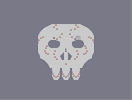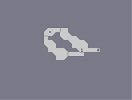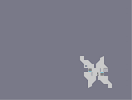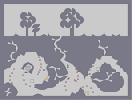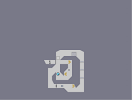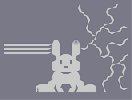The Cold of Death Ring Around the Rosie A Few Good Reasons to Get Health Insurance Jack, Be Nimble The Hamster Bunny Tunes

Pages: (0)

### For the record

I wasn't hating on the map, it's pretty cool.

### "...an obvious bad but maybe a little bit humourous one."### wow

really remvoed that?

WHO IS DELETING ALL OF THE COMMENTS

### goddamn

i could really do with some waffles.

### Oh, come on.

What the hell is it with all the hate for this map?

I know my review is shit and I didn't even try to make a good one but the map doesn't deserve the frustration.

I try my best to review at least one map per month but I was very busy in the last weeks and wasn't able to find new feature-worthy maps and write a nice review. So... my best idea was to write an obvious bad but maybe a little bit humourous one. I guess I failed hard.

### I liked it.

Pretty cool, however simple it may be.
Any input always appreciated.

### .

Okay maybe stagnation was a bit harsh. The featured list had been flowing quite well until this blip, and it's good to hear the immediate future is covered. I realise it's not high on anyone's agenda, it just seemed a little crowdsourcing could solve the problem. I guess the 23 replies over the last couple of years on that thread puts that idea to rest though. I will submit a couple of quick reviews anyway, no harm done.
First of all -- there's no "stagnation of the featured maps list" at the moment. We've got the next ten spots or so covered; I can't help that people occasionally forget this unimportant part of their lives.

Second, we stopped advertising for the Creature Feature thread (link below courtesy of 1211) because its use died down. But the unused reviews are still all there. And it's still open to submissions. So go for it.

### There's a thread for that on the forums.

Creature Feature [ninjarobotyeti.com]

You can post your reviews here and it will plausibly be featured.

### Mammaletto's idea is brilliant

If such a system isn't implemented soon, expect this site to settle at the bottom of the septic tank of cyberspace.

### A question for reviewers / admins

Would it be possible to introduce a system whereby non-reviewers submit reviews to reviewers and they are screened and / or edited? Reviewers review non-reviewers' reviews. And by review I mean feature. Would end the stagnation of the featured maps list, no?

### douche move

mintnut have a point
This map is brilliant, dc. Not sure how I missed it. 5aved.

### The problem with this

is probably that the reviewer isn't considering the way he is treating the author whose map he's featuring. Sure it's nice to be on the front page, but when you brazenly accompany it with the whole "here's just any old map, since I'm busy/in a rush/out of good maps" it kind of ruins the sincerity. People deserve to have their maps featured on merit and with respect.

Point is, you've drawn far more attention to the lack of feature, and caused far more ill feeling than if you'd just left the last one up for longer. You could have just written a normal review for this and everything would have been fine.
llabesab, you haven't been around numa at all and you comment like this?

also features are up to the reviewer if you don't like the review, might I suggest looking through some of the 222520+ maps archived here?
And so the decline of NUMA begins...

### Virtually the entire review screams "lazy" and "shoddy".

I don't see much aesthetic value in the map either, but really it's subjective and doesn't bother me too much.

### There's a certain n-art which really deserves to be featured.

Oldies know what I'm talking about.

..I guess.
Awesome indeed.

and thanks :)

### hey mate

they are soo fitt need to contact 1211 now to see if he likes em too

### im sorry but no computer busted

i was thinking if apakanua doesnt do the tiles how about these? ---- 000000011000N100011111100000000P00N10001111111000000010011101111111010000003100190050001500100000150001@000000000N10000021100N100310400011000000000000015N01111110000000140000100000Q>11000000010001@14N10O0?1100000035000100210N11111000000103P?1400000Q100100000021501A210000000310000000000100001100015000000000001@0G00240010>0000000000>11E0001002400000000K1400000031P00110000000100000000Q11P00Q0000000110001140000000?00000003500350000000K11000000010301000010011110000000115014000P01501100000000000Q11011010002000000000000>10Q10240O00000000031G000001A01110000000015NM0?1D000000000000000100001100G1A3103000000014N10NM0111011110000000021001000N102P0>0000000000000?1001@N11000000000000001I0000000000000000000001H000000?1|

buddy :)

### yeah

i need an update to from apakanua who is currently on tiles he gave me a half piece of work but we decided he is better of doing a whole tile set before we start making some changes.